DownloadDocumentationHNS → Program for filter transfer function forming

## Program for filter transfer function forming with examples

Transfer function of recursion digital filter is the ratio of two polynomials of degreefrom pahse shift operator:(1)
Traditionally in digital filters polynomials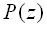and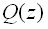have real or complex coefficients. Let us assume that these coefficients are numbers belonging to some hypercomplex numerical systemof dimension. Let us convert the transfer function (1) with hypercomplex coefficients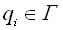to the form of hypercomplex function of such kind: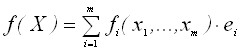,
where: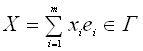;,- basis elements of hypercomplex number system.

For such transformation, numerator and denominator (1) need to be multiplied on such hypercomplex number, that in the denominator hypercomplex number of such kind.wil be received,
where:- real number;- unit element of hypercomplex numerical system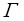.
Such multiplier is number adjoined to number, i.e.,, andis a norm of hypercomplex number, i.e.,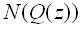. The norm of hypercomplex number is a form of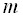-th dimension from this number components. Asis polynomial of degree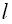, normis a form of degreefrom-operator.
Accordingly, adjoined numberwill be form of degree, and productwill be form of degreefrom elements of hypercomplex number, and form of degreefrom-operator.
Thus, transfer function (1), but with hypercomplex coefficients, will turn into such one: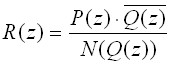,                                (2)
which can be presented as a hypercomplex function:.                    (3)
Each component of this hypercomplex function can be considered as some transfer function of filter orderwith real coefficients.
Work of the filters is described by recurrent equation:.

Signal on the input of hypercomplex filter with such structure is real, but after the first multiplying on one of coefficients of filter it turns into hypercomplex one. So, only hypercomplex values circulate inside the filter.
Filter output is hypercomplex. But only one of channels, transfer function on which coincides with the given one, is used.
At hypercomplex filter structure modeling, it is possible to use any hypercomplex systems. But it is necessary to take into account that norms in some hypercomplex numerical systems are of a very simple form, and that is why they will contain very little amount of hypercomplex number components as parameters.
Let us consider, as an example, the synthesis of structure of concrete third order filter with the help of triplex system of hypercomplex numbers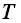.
Formula of triplex numbers multiplying: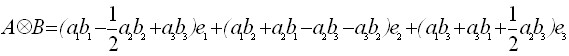,  (4)
and expression for adjoined number in the triplex system of numbers is:.          (5)
Let us assume that transfer function of 3th order filter with real coefficients, and its corresponding equation have the following form:;                                                    (6)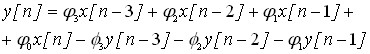,
and transfer function of the 1st order filter with triplex coefficients and its corresponding equation are:;                          (7).
Let convert transfer function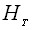into the form (3). Let us define the norm of denominator:.                  (8)
By equating coefficients at the identical degrees in denominator (6) and norm (8), we receive the system of equations, solutions of which give triplex coefficient: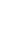.       (9)
The numerator of expression (7) is defined with (8) and (10):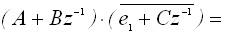(10)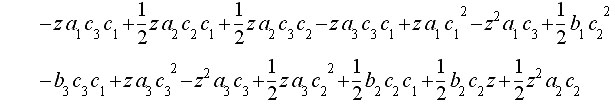If we put (8) and (10) to right part of (3), we will receive hypercomplex function, each of three components of which can be used as filter transfer function. Let us use the first component of this hypercomplex function (7). By equating coefficients at the identical degrees at-shift operator in numerator of this component and transfer function (4.6), we will receive system of equations with 4 linear equations from 6 unknowns:(it will be recalled that unknownswere received before from system (9)). Therefore, two unknowns are free. Unknownsand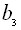, which are more frequently included in equations of the system – 6 and 4 times, accordingly, are expedient to be taken as free unknowns. For simplification, let these free unknowns equal zero: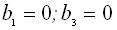.                                 (11)
Taking this into account, system of equations for the calculation of other components of hypercomplex coefficients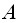andwill be:(12)
Thus, components of triplex coefficientsof hypercomplex filter of 1st order (7), which is equivalent to filter of the 3rd order with real coefficients (6), is determined by solutions of systems (9) and (12) with regard to (12).
Work of triplex filter comes to the recurrent calculations after equation (7), basic actions in which are multiplication and addition of triplex numbers corresponding to formula (4), which includes 10 multiplications and 7 additions of real numbers. To reduce the amount of operations, it is advisably to transfer data and coefficients from system of triplex numbersto system. If in systemthe number has the following form:, than in systemit will turn into the following:and multiplying formula inwill be the following:that is considerably simpler than formula (4).
Return transfer from systemto system of triplex numbers can be made using formula:.
Let us consider the example of certain elliptic filter of low frequencies of 3rd order realization. Transfer function with real coefficients is the following:.           (13)
The gain-frequency characteristic is shown on fig. 1.
If the gain-frequency characteristic is expressed in form (6), then the triplex coefficient is:.
System (9) with such right parts has 6 solutions, 2 of which are real. Using one of them and solving system (2) by the help of (1), we will receive such coefficients of triplex transfer function (7):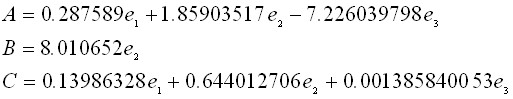• The gain-frequency characteristic of the first component of this transfer function fully coincides with the gain-frequency characteristic of filter with real coefficients shown on fig. 1.
To model the work of both filter variants, the same discrete signal is sent to their inputs. This signal is the mix of two sinusoids. Their frequencies are selected so that higher frequency must be filtered, and lower, no:• The input signal is shown on fig. 2.
• The signal at output of 3rd order filter with real coefficients (2) is shown on fig.3.
• Output signals after filtration with triplex coefficients filter are shown on fig.4-6.
Comparison of fig.3 and fig.4 testify that processes of acceleration of filter with real coefficients and triplex filter differ a little, but in a steady-state regime they work identically, selecting lower frequency from the input signal. Two other outputs do not carry useful information, but they are needed to form the output signal on the first output.Fig.1. Frequency response of 3rd order filter with real coefficients and 1st order filter with hypercomplex coefficients.Fig.2. Input signal.Pic. 3. Output signal after filtration with use of 3rd order filter with real coefficients.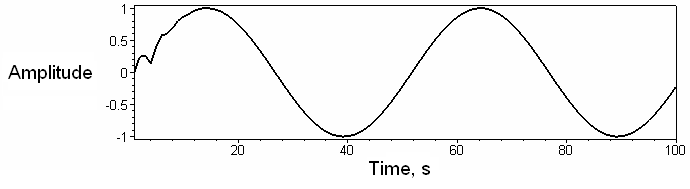Fig. 4. Output signal on the first output of triplex filter.Fig. 5. Output signal on the second output of triplex filter.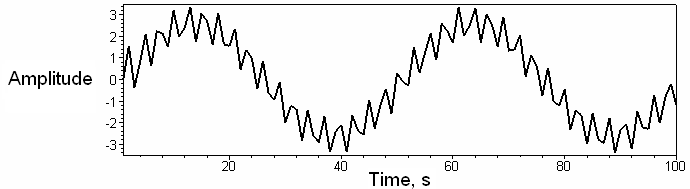Fig. 6. Output signal on the third output of triplex filter.